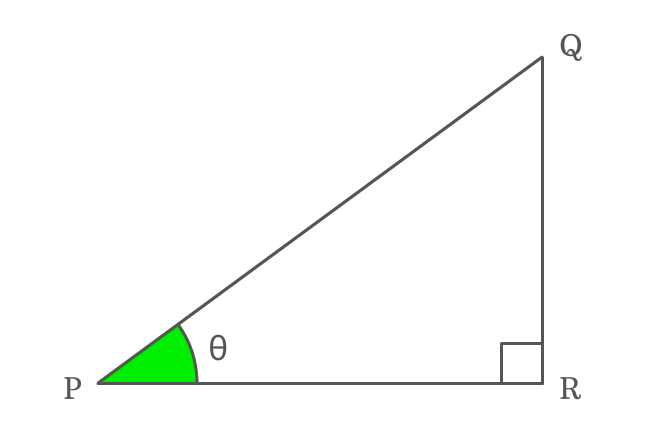# Reciprocal identity of Tan function

## Formula

$\cot{\theta} \,=\, \dfrac{1}{\tan{\theta}}$

### Proof

Tangent is a ratio of lengths of opposite side to adjacent side and the cotangent is a ratio of lengths of adjacent side to opposite side. The tan and cot functions are mutual reciprocal functions. Hence, the reciprocal of tan of angle is equals to cot of angle.

$\Delta RPQ$ is a right triangle and its angle is assumed as theta.#### Write Tangent in its ratio form

Write tan of angle theta ($\tan{\theta}$) firstly in its ratio form.

$\tan{\theta} \,=\, \dfrac{QR}{PR}$

#### Write cotangent in its ratio form

Similarly, express the cotangent of angle theta ($\cot{\theta}$) in its ratio form.

$\cot{\theta} \,=\, \dfrac{PR}{QR}$

#### Relation between Tangent and Cotangent

Now, write the value in the form of ratio of cot function in reciprocal form to derive the relation between tan and cot functions in mathematics.

$\implies \cot{\theta} \,=\, \dfrac{1}{\dfrac{QR}{PR}}$

$\,\,\, \therefore \,\,\,\,\,\,$ $\cot{\theta} \,=\, \dfrac{1}{\tan{\theta}}$

Therefore, it is successfully proved that the reciprocal of tan function equals to cot function. It is used as a formula in trigonometry.

###### Note

The angle of a right triangle can be represented by any symbol but the reciprocal identity of tan function should be expressed in the corresponding angle.

For example, if $x$ denotes angle of right triangle, then

$\cot{x} \,=\, \dfrac{1}{\tan{x}}$

In the same way, if $A$ represents angle of right triangle, then

$\cot{A} \,=\, \dfrac{1}{\tan{A}}$

The reciprocal identity of tan function is written in this form but the symbol of angle of right triangle is changed.

Latest Math Topics
Jun 26, 2023
Jun 23, 2023

Latest Math Problems
Jul 01, 2023
Jun 25, 2023
###### Math Questions

The math problems with solutions to learn how to solve a problem.

Learn solutions

Practice now

###### Math Videos

The math videos tutorials with visual graphics to learn every concept.

Watch now

###### Subscribe us

Get the latest math updates from the Math Doubts by subscribing us.